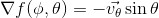#1

I assume the gradient of cosine iswhere the vector v is spherical unit vector on sphere surface pointing to direction opposite z axis. I was expecting to see this vector instead of vk(base plane unit vector) in the book. Are they same?